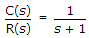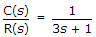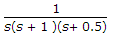# Electronics and Communication Engineering - Automatic Control Systems

Exercise : Automatic Control Systems - Section 7
1.
Consider the following systems
System I,System II,where C(s) is the output and R(s) is the input of these systems
System I has larger bandwidth and faster speed of response than System II
System II has larger bandwidth and faster speed of response than System I
System I has larger bandwidth but System II has faster speed of response
System II has larger bandwidth but System I has faster speed of response
Explanation:
No answer description is available. Let's discuss.

2.
Nyquist stability criterion is based on a theorem from the theory of complex variables.
True
False
Explanation:
No answer description is available. Let's discuss.

3.
For the function G(s) H(s) =the phase cross-over frequency is
Explanation:
No answer description is available. Let's discuss.

4.
While forming Routh's array, the situation of a row of zeros indicates that the system
has symmetrically located roots
is not sensitive to variations in gain
is stable
is unstable
Explanation:
No answer description is available. Let's discuss.

5.

Assertion (A): In liquid system the ratio of change in liquid level to change in flow rate is called resistance of liquid flow.

Reason (R): In liquid level systems the ratio of change in liquid stored in a tank to the change in head is called capacitance of tank.

Both A and R are correct and R is correct explanation of A
Both A and R are correct but R is not correct explanation of A
A is correct but R is wrong
R is correct but A is wrong